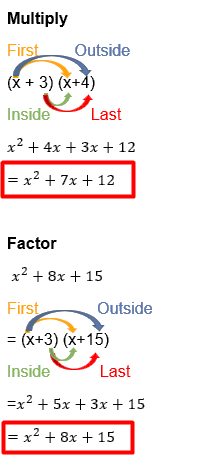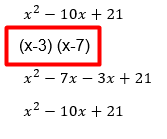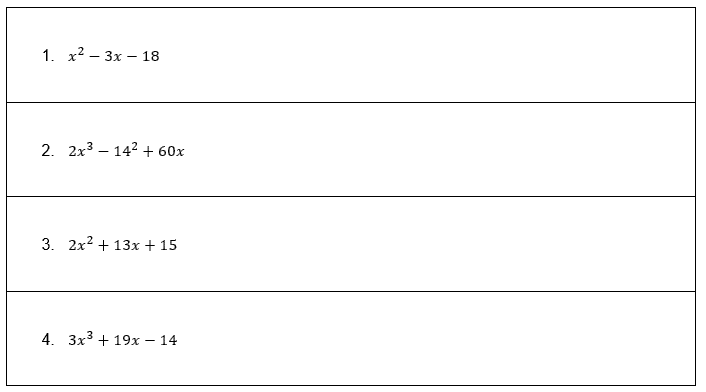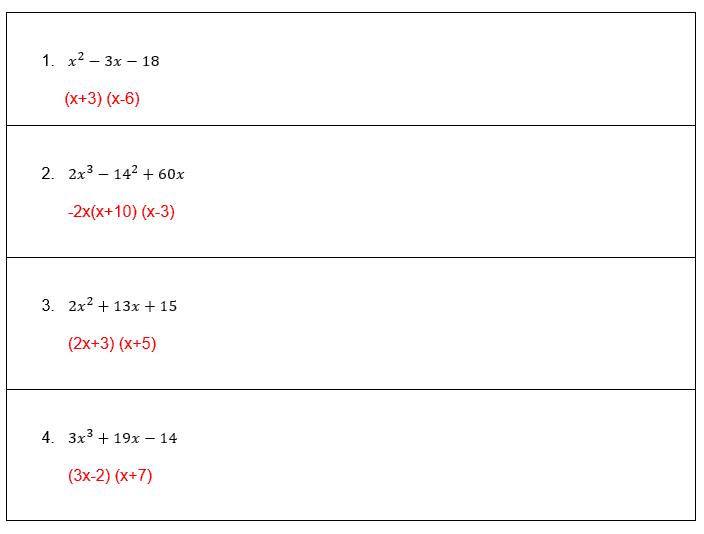# Factoring Trinomials Guess and Check Worksheet (with answer key + PDF)

Polynomials with three terms are referred to as trinomials. When an expression is factored, it is written as the result of at least two other expressions. One of two types of squares can describe a trinomial: perfect and imperfect.

## What are the “Factoring Trinomials Guess and Check Worksheet”?

This worksheet will explore some of the Factoring Trinomials Guess and Check.

## What is Factoring Trinomials Guess and Check?

A polynomial with three terms is referred to as a trinomial. An x term appears the first time, followed by an x term the second time, and a constant the third time (just a number)

This worksheet will help the student to understand and solve the different difficult questions using the FOIL method.

## Instructions on how to use the “Factoring Trinomials Guess and Check Worksheet.”

Study the concept and examples given and try to solve the given exercises below.

## Conclusion

The “Guess and Check” method’s strategy is to make an educated guess at a solution, then plug that guess back into the issue to see if you came up with the right response. If the answer is off, try again with a guess that will bring you closer to the objective, and keep guessing until you find the right answer.

If you have any inquiries or feedback, please let us know.

## Factoring Trinomials Guess and Check Worksheet (with answer key + PDF)

Example:Example:## Worksheet

1. Solve the following.1. Reflection

Was the lesson challenging or simple to understand?